## Example Questions

### Example Question #1 : Rectangles

A rectangle has an area of 48 and a perimeter of 28.  What are its dimensions?

2 x 24

0.25 x 192

1 x 48

6 x 8

16 x 3

6 x 8

Explanation:

We can set up our data into the following two equations:

(Area) LH = 48

(Perimeter) 2L + 2H = 28

Solve the area equation for one of the two variables (here, length): L = 48 / H

Place that value for L into ever place you find L in the perimeter equation: 2(48 / H) + 2H = 28; then simplify:

96/H + 2H = 28

Multiply through by H: 96 + 2H2 = 28H

Get everything on the same side of the equals sign: 2H2 - 28H + 96 = 0

Divide out the common 2: H2 - 14H + 48 = 0

Factor: (H - 6) (H - 8) = 0

Either of these multiples can be 0, therefore, consider each one separately:

H - 6 = 0; H = 6

H - 8 = 0; H = 8

Because this is a rectangle, these two dimensions are the height and width.  If you choose 6 for the "height" the other perpendicular dimension would be 8 and vice-versa.  Therefore, the dimensions are 6 x 8.

### Example Question #2 : Rectangles

The length of a rectangle is three times its width, and the perimeter is. What is the width of the rectangle?Explanation:

For any rectangle,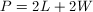, where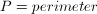,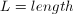, and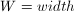In this problem, we are given that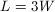(length is three times the width), so replace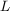in the perimeter equation with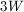: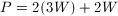Plug in our value for the perimeter,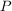: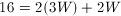Simplify: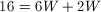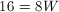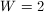### Example Question #3 : Rectangles

The area of a rectangle is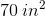. Its perimeter is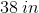. What is the length of its shorter side?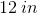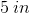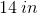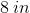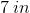Explanation:

We know that the following two equations hold for rectangles. For area: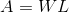For perimeter: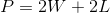Now, for our data, we know: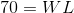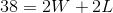Now, solve the first equation for one of the variables: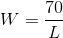Now, substitute this value into the second equation: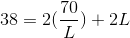Solve for: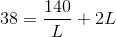Multiply both sides by: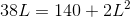Solve as a quadratic. Divide through by: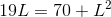Now, get the equation into standard form: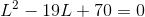Factor this: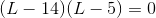This means that(or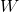) would equal eitheror. Therefore, your answer is.

Tired of practice problems?

Try live online GRE prep today.